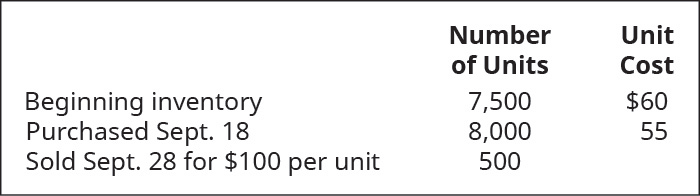# Use the weighted-average (AVG) cost allocation method, with perpetual inventory updating, to calculate (a) sales revenue, (b) cost of goods sold, and c) gross margin for B75 Company, considering the following transactions.FindFindarrow_forward

### Principles of Accounting Volume 1

19th Edition
OpenStax
Publisher: OpenStax College
ISBN: 9781947172685

#### Solutions

Chapter
SectionFindFindarrow_forward

### Principles of Accounting Volume 1

19th Edition
OpenStax
Publisher: OpenStax College
ISBN: 9781947172685
Chapter 10, Problem 7PB
Textbook Problem
1 views

## Use the weighted-average (AVG) cost allocation method, with perpetual inventory updating, to calculate (a) sales revenue, (b) cost of goods sold, and c) gross margin for B75 Company, considering the following transactions.To determine

(a)

Concept introduction:

Perpetual Inventory System:

The perpetual inventory system records and updates the inventory after each and every transaction. The inventory balance is updated after each transaction and it is kept up to date at every time.

Weighted Average method:

Under this method, the cost per unit of the inventory is calculated as weighted average cost per unit and the cost of goods sold and inventory is calculated with the help of weighted average cost per unit.

To indicate:

Sales revenue

### Explanation of Solution

 Number of Units Unit Rate Total
To determine

(b)

Concept introduction:

Perpetual Inventory System:

The perpetual inventory system records and updates the inventory after each and every transaction. The inventory balance is updated after each transaction and it is kept up to date at every time.

Weighted Average method:

Under this method, the cost per unit of the inventory is calculated as weighted average cost per unit and the cost of goods sold and inventory is calculated with the help of weighted average cost per unit.

To indicate:

Cost of goods sold

To determine

(c)

Concept introduction:

Perpetual Inventory System:

The perpetual inventory system records and updates the inventory after each and every transaction. The inventory balance is updated after each transaction and it is kept up to date at every time.

Weighted Average method:

Under this method, the cost per unit of the inventory is calculated as weighted average cost per unit and the cost of goods sold and inventory is calculated with the help of weighted average cost per unit.

To indicate:

Gross Margin

### Still sussing out bartleby?

Check out a sample textbook solution.

See a sample solution

#### The Solution to Your Study Problems

Bartleby provides explanations to thousands of textbook problems written by our experts, many with advanced degrees!

Get Started

Find more solutions based on key concepts
STATEMENT OF CASH FLOWS W.C. Cycling had 55,000 in cash at year-end 2013 and 25,000 in cash at year-end 2014. T...

Fundamentals of Financial Management, Concise Edition (with Thomson ONE - Business School Edition, 1 term (6 months) Printed Access Card) (MindTap Course List)

How did Taylor change management?

Principles of Management